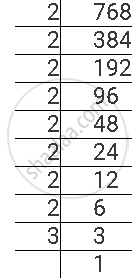Share

# For the Following Number, Find the Smallest Whole Number by Which It Should Be Multiplied So as to Get a Perfect Square Number. Also Find the Square Root of the Square Number So Obtained. 768 - CBSE Class 8 - Mathematics

ConceptSquare Roots - Finding Square Root Through Prime Factorisation

#### Question

For the following number, find the smallest whole number by which it should be multiplied so as to get a perfect square number. Also find the square root of the square number so obtained.

768

#### Solution

768 can be factorised as follows.768 = 2 × 2 × 2 × 2 × 2 × 2 × 2 × 2 × 3

Here, prime factor 3 does not have its pair. If 3 gets a pair, then the number will become a perfect square. Therefore, 768 has to be multiplied with 3 to obtain a perfect square.

Therefore, 768 × 3 = 2304 is a perfect square.

768 × 3 = 2304 = 2 × 2 × 2 × 2 × 2 × 2 × 2 × 2 × 3 × 3

∴ sqrt(2304) = 2 x 2 x 2 x 2 x 3 = 48

Is there an error in this question or solution?

#### APPEARS IN

NCERT Solution for Mathematics Textbook for Class 8 (2018 to Current)
Chapter 6: Squares and Square Roots
Ex. 6.30 | Q: 5.6 | Page no. 102
Solution For the Following Number, Find the Smallest Whole Number by Which It Should Be Multiplied So as to Get a Perfect Square Number. Also Find the Square Root of the Square Number So Obtained. 768 Concept: Square Roots - Finding Square Root Through Prime Factorisation.
S